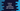# Python range explanation with example### Python range() explanation with example :

In this tutorial, we will learn python range() function and how to use it using examples. Mainly, range() is used in for loops. It can be defined as below :

``````range(stop)
or
range(start, stop[,step])``````

### range(stop) :

range(stop) will create one list from 0 to stop - 1 and returns one iterator for that list. We can use the iterator to traverse through the list. Following example explains how to iterate through a list create using range :

``````for i in range(10):
print(i)``````

It will produce the following result :

``````0
1
2
3
4
5
6
7
8
9``````

But remember , it doesn’t returns one list. Check the below example :

``````my_list = [1,2,3,4,5]
my_range = range(5)

print("Type of my_list : ",type(my_list))
print(my_list)

print("Type of my_range : ",type(my_range))
print(my_range)``````

It will print the following output :

``````Type of my_list :  <class 'list'>
[1, 2, 3, 4, 5]
Type of my_range :  <class 'range'>
range(0, 5)``````

So , the type is range, not list.

### Create a list using range():

We have seen that range() doesn’t create one list. But we can create a list using it like below :

``````my_list = list(range(5))

print("Type of my_list : ",type(my_list))
print(my_list)``````

Output is :

``````Type of my_list :  <class 'list'>
[0, 1, 2, 3, 4]``````

### Traversing a list using python range() :

Since range(n) returns one iterator to iterate from 0 to n-1 . So, if we use range(length(list)) , it will allow us to iterate from 0 to length of the list - 1 . Using this technique, we can iterate all elements of a list as below :

``````my_list = [1,2,3,4,5]

for i in range(len(my_list)):
print(my_list[i])``````

It will give the following output :

``````1
2
3
4
5``````

### Examples of range(start, stop[,step]) in python :

We have seen above how range(stop) works and how to use it to traverse a for loop in python. range(start,stop[,step]) is also similar as above. It returns one iterator and we can use it to iterate through elements of a for-loop.

start means the starting value for the iterator. end means the ending value for the iterator. step is optional, it means step size for the iterator. For range(n), start is 0 and end is n.

### Example of range with start and stop :

``````for i in range(5,10):
print(i)``````

It will print :

``````5
6
7
8
9``````

Here ,start is 5 and end is 10

### Example of range with start, stop and step :

``````for i in range(5,10,2):
print(i)``````

It will print :

``````5
7
9``````

This is same as above . start is 5, end is 10. But, we have added one more parameter 2 which works as step . That’s why one element is skipped each time.

### Iterate a sub list using range :

We can use range(start, end) to iterate through a sub-list of a list from start index to end.

``````my_list = [1,2,3,4,5,6,7,8,9,10]

for i in range(5,10):
print(my_list[i])``````

Output :

``````6
7
8
9
10``````

In the same way, we can iterate through a list with a step between the elements :

``````my_list = [1,2,3,4,5,6,7,8,9,10]

for i in range(3,10,2):
print(my_list[i])``````

It will print :

``````4
6
8
10``````

It is same as above example, only 2 is used as step. So, it prints alternate numbers.